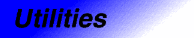# EqualityComparableCategory: utilities Component type: concept

## Description

A type is EqualityComparable if objects of that type can be compared for equality using `operator==`, and if `operator==` is an equivalence relation.

## Notation

 `X` A type that is a model of EqualityComparable `x`, `y`, `z` Object of type `X`

## Valid expressions

Name Expression Type requirements Return type
Equality `x == y`   Convertible to `bool`
Inequality `x != y`   Convertible to `bool`

## Expression semantics

Name Expression Precondition Semantics Postcondition
Equality `x == y` `x` and `y` are in the domain of `==`
Inequality `x != y` `x` and `y` are in the domain of `==` Equivalent to `!(x == y)`

## Invariants

 Identity `&x == &y` implies `x` == `y` Reflexivity `x == x` Symmetry `x == y` implies `y == x` Transitivity `x == y` and `y == z` implies `x == z`

## Models

• int
• `Vector<int>`